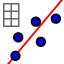# IM1.8.7 Residuals 1

Author:
Lind, Jon

## Plotting the residuals

Below is your data from lesson 8.5 with the CC values for each set. 1. Use Two Variable regression analysison Set F (the set with the "best" positive CC). 2. Below the scatterplot, choose a Linear Regression to get the LOBF. Pretty good, right? 3. Above the scatterplot, change to Residual Plot. What do you see? 4. Test the other data sets, keeping in mind their CC values, and try to figure out what a residual plot shows.

1. What information is shown on a residual plot? 2. How is a residual plot related to the CC? 3. What does a residual plot look like for data that is a "good fit" for a linear model? 4. What does a residual plot look like for data that is a "bad fit" for a linear model?